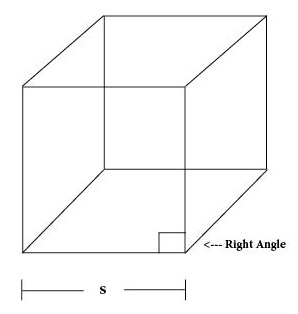# Study Tips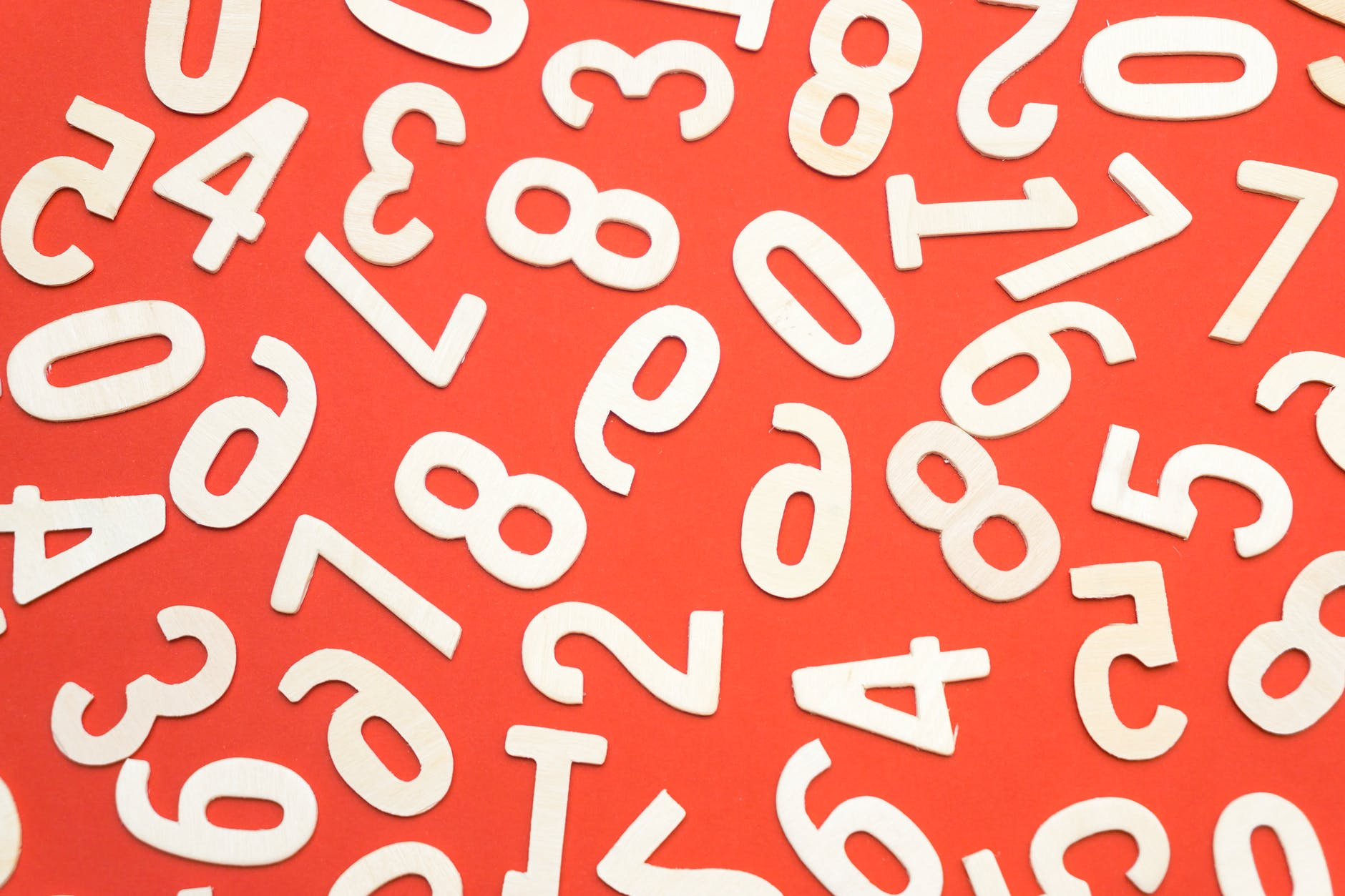# More Extra Math Questions# Extra Math Questions# Distance# Total Cost# Point-Slope

Point-Slope Form of the Equation of a Line
point-slope form of the equation of a line
y – y1 = m(x – x1)

# Number Sense# Power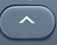# Root# Scientific Notation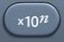# Decimals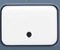# Measurement

1 foot = 12 inches

1 meter = 100 centimeters

# Toggle# π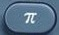# Negatives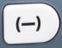# Combo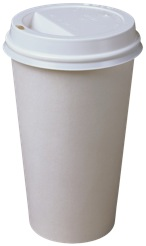# Probability# Fractions# Order of Operations

Order of Operations = PEMDAS

# Calculator# Slope-Intercept# Slope

Slope refers to the Steepness of a Line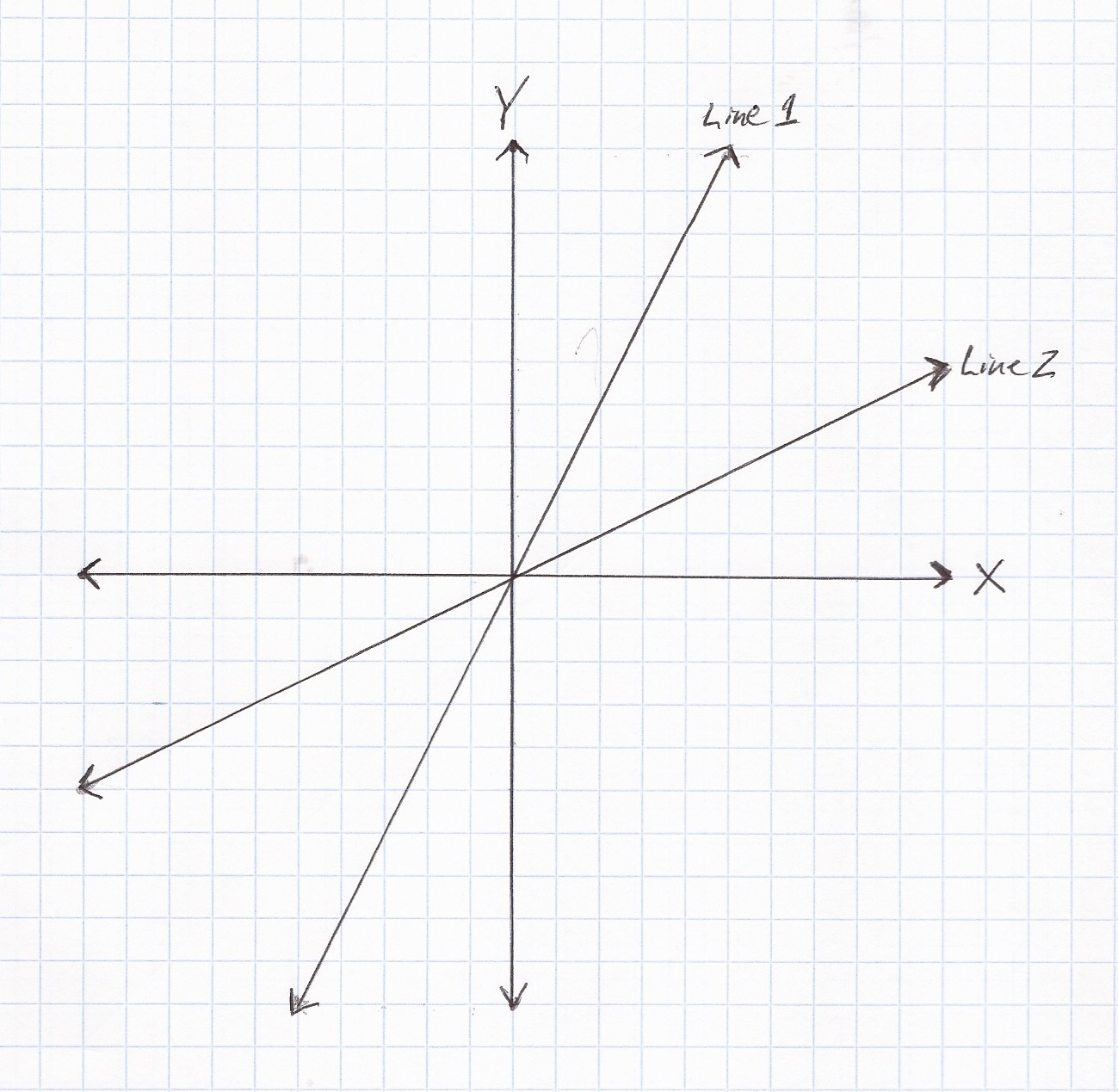# Line# Inequality Plotting

x > 5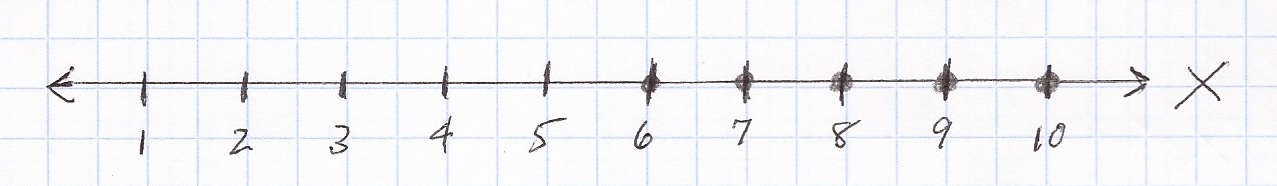x ≥ 5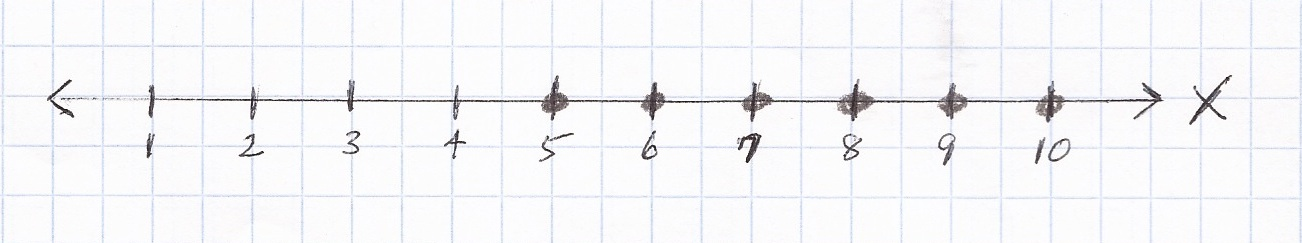# Inequalities

<                              ≤                              >                              ≥

# Plug-In# Pythagorean Theorem

### a²  +  b²  =  c²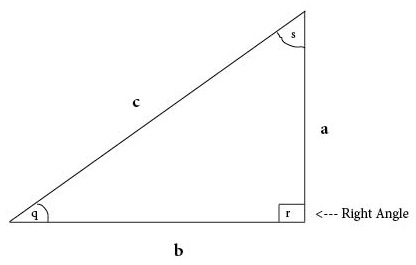# Simple Interest# Parallelogram

Parallelogram = Flat Four-Sided Shape in which Opposite Sides are Parallel

# Trapezoid

Trapezoid = Flat Four-Sided Shape with Two Parallel Sides

# Cylinder

Cylinder = Three-Dimensional Shape with Circular Top and Base# Right Rectangular Prism

Right Rectangular Prism = Three-Dimensional Shape in which Opposite Faces are Equal (with all right angles)# Pyramid

Pyramid = Three-Dimensional Shape with Polygonal Base Connecting to a Point# Sphere

Sphere = 3-Dimensional Round Shape with All Surface Points Equidistant from Center# Cone

Cone = Three-Dimensional Shape with Circular Base Connecting to a Point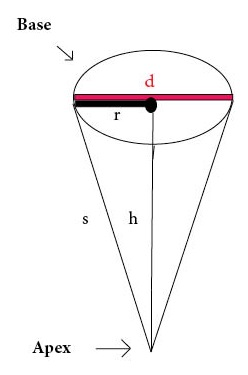# Mode

Mode = Most Frequent Number

# Mean

Mean =$\bf\displaystyle\frac{Sum}{Count}$

# Triangle

Triangle = Flat Shape with Three Sides# Rectangle

Rectangle = Flat Shape with Four Sides (with four right angles)

# Cube Root

Cube Root = ³Number³ = ³Number × Itself × Itself

# Cubing3

Number Cubed = Number × Itself × Itself = Number³

# Cube

Cube = Three-Dimensional Shape with Six Square Faces (with all right angles)